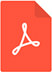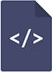Time-frequency analyses applied to sound brought about by continual impacts for time-varying axial stress measurement of round barPDF Full Text

Abstract

We developed the method to measure a time-varying stress of a round bar by time-frequency analyses. The larger tension we give to a guitar string, the higher tone the string emits, i.e. the string is changing its natural frequency to be higher. The frequency of the string is a function of the tension. When we look at the relation conversely, we can estimate tension by the frequency. We conceived the idea that if we could measure the variation of the frequency, we would be able to evaluate how the tension varied with time. The same condition holds for a round bar. We are able to estimate the time-varying axial stress of the bar by measuring the variation of the natural frequency of the bar. In order to verify of this idea, we conducted the experiment. We applied a sinusoidal stress to the bar. We gave continual impacts to the bar to bring about free vibrations. The vibrations produce sound. The sound provides data to follow the variation of the natural frequency. The procedure which consisted of a short-time Fourier transform application and signal processing programs we developed was applied to the sound. We could obtain the sequence of points which described the relation between the first mode natural frequency of the bar and time. We converted the sequence of the frequency to the sequence of the axial stress employing the relation between the natural frequency and the axial stress of the bar. We compared the stress sequence estimated by the procedure with the stress variation measured by the strain gauge attached to the bar. The sequences by the procedure and the variation by the strain gauge agreed well for various sinusoidal applied stresses for the loading cycle of less than 10 Hz.

Keywords

short-time fourier transform, time-varying stress, sound, natural frequency, round bar